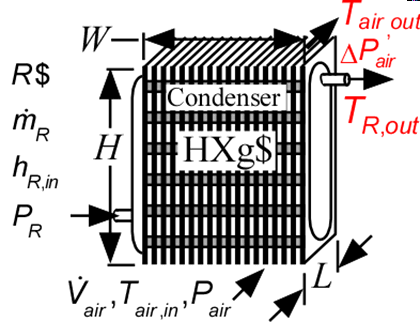Contents - index

Condenser4_CLCondenser4_CL determines the outlet state of an air-cooled finned circular tube condenser given the inlet states of the air and refrigerant and the heat transfer surface area.  Thermal energy is rejected to an air stream.  It is necessary to provide the dimensions of the condenser and the type of finned circular surface, chosen from the list of compact heat exchangers.   The heat transfer coefficients are internally determined using the functions in the Heat Transfer Library See Condenser2_CL which provides the same capability, except that the heat transfer coefficients are provided to the routine, rather than internally calculated.  Use Condenser3_CL to determine the length in the flow direction for a given frontal area at design conditions.

Inputs:

R\$:   name of the refrigerant

m_dot_R:  refrigerant flow rate (kg/s, lbm/hr)

h_R_in:  specific enthalpy of entering refrigerant (J/kg, kJ/kg, Btu/lbm)

P_R:  refrigerant pressure (Pa, kPa, bar, MPa, psia, atm)

V_dot:  volumetric flow rate of air (m^3/s, cfm)

T_air_in:  air inlet temperature  (C, K, F, R)

P_air:  air pressure (Pa, kPa, bar, MPa, psia, atm)

HXg\$:  string identifier for a finned circular heat exchanger in the compact heat exchanger geometry

W:   width of heat exchanger face parallel to tubes (m or ft)

H:  height of heat exchanger face perpendicular to tubes (m or ft)

L:   length of heat exchanger in flow direction (m or ft)

th_tb:  tube thickness (m or ft); if set to a negative number then a reasonable value is assumed

N_circuits:  number of parallel flow circuits for the refrigerant

Outputs:

Q_dot:  overall heat transfer rate (W, kW, Btu/hr)

h_R_out: refrigerant outlet specific enthalpy (J/kg, kJ/kg, Btu/lbm)

T_air_out:  outlet temperature of the air  (C, K, F, R)

DELTAP_air: pressure drop on air side (Pa, kPa, MPa, bar, psi, atm)

Example:

\$unitSystem SI C kPa kJ mass

\$TabStops 0.2 4.5 in

R\$='R134a' "refrigerant"

m_dot_R=0.0028 [kg/s] "refrigerant mass flow rate"

P_R=1 [MPa]*convert(MPa,kPa) "refrigerant pressure"

h_R_in=enthalpy(R\$,T=60 [C],P=P_R) "specific enthalpy of entering refrigerant"

V_dot_air=0.06 [m^3/s] "volumetric flow rate of air"

T_air_in=20 [C] "air inlet temperature"

P_air=1 [atm]*convert(atm,kPa) "air pressure"

HXg\$='fc_tubes_s80-38T'

th = 0.9 [mm]*convert(mm,m) "tube wall thickness"

W=0.2 [m] "length of tubes"

H=0.2  [m] "height of flow channel"

N_circuits=1 "number of parallel circuits"

L=0.057

Call condenser4_cl(R\$, m_dot_R, h_R_in, P_R, V_dot_air, T_air_in, P_air, HXg\$, W, H, L, th, N_circuits :  Q_dot, h_R_out, T_air_out, DELTAP_air)

{Solution:

DELTAP_air=0.009808

h_R_out=105.5

Q_dot=0.526

T_air_out=27.25

}